# Model Predictive Control for Closed-Loop Insulin Delivery¶

(Or: how I learned to stop worrying and replaced my daughter's pancreas with TensorFlow)

Marius Eriksen (@marius, [email protected]), March 2018.

People with Type-1 Diabetes do not have a functioning pancreas, the organ responsible for regulating insulin levels in your body. Insulin is a hormone that regulates the metabolism of glucose (and also fats and proteins); it works by signaling, among others, fat and liver cells to absorb glucose. Blood glucose needs to remain in a fairly narrow "Goldilocks" zone: high levels of blood glucose can have severe long-term health consequences; low levels of blood glucose can lead to unconsciousness or even death.

Without a functioning pancreas, people living with Type-1 Diabetes must assume its job. Insulin must be provided when blood glucose is high (it is usually injected subcutaneously with a syringe or an insulin pump), but not too much or blood glucose levels will go too low. (If glucose levels do go low, fast-acting carbohydrates must be quickly consumed to counteract the effects of the excess insulin.) Typically, people with Type-1 Diabetes deliver insulin through an insulin pump or through subcutaneous injections.

What's more, whereas the pancreas can secrete insulin directly into the blood stream, so that cells are signaled with little delay, externally administered insulin takes much longer to reach its destination. FiASP is the fastest acting subcutaneously delivered insulin on the market today, and while it enters the blood stream quite quickly, its maximum effect on blood glucose is between 1 and 3 hours after injection. This hints at yet another complication: the pharmacokinetics of insulin analogs like used to treat Type-1 Diabetes. After a unit of insulin is delivered, it trickles into the blood stream over the course of many hours. The following shows how the concentration of FiASP evolves in blood for the first two hours after an injection.Carbohydrates consumed in food, by the way, has its own kinetics. Depending on the amount of carbohydrates and the food's glycaemic index, a meal can take anywhere between an hour or six to fully digest: the carbohydrates in the meal enter the blood stream over the same course of time.

And these are far from the only factors that affect blood glucose! Stress levels, exercise, diurnal rhythms, and illness all have a significant effect on how your body metabolizes sugar, or how your cells respond to insulin. So too do imperfections in insulin manufacturing, the site at which insulin is injected, the temperature at which insulin is kept, and even the mechanical design of an insulin pump.

It's easy to see how Type-1 (T1D) diabetes is a therapy intense disorder. People living with T1D must constantly adjust insulin delivery (or drink some juice), depending on any of the above factors. People with T1D must also anticipate meals and estimate the amount of carbohydrates that they will eat. Ideally, they inject insulin in good time before starting a meal so that the insulin has time to enter the blood stream in sufficient quantities. (But don't wait too long, or your blood glucose will go too low!)

Closed-loop insulin delivery systems, or "artificial pancreases" are designed to assume much of this burden. Such systems automate insulin delivery in order to maintain "Goldilocks-zone" blood glucose levels. These systems read glucose levels from a continuous glucose monitor and make regular insulin deliveries through an insulin pump based on current and predicted glucose levels.

We describe a model for predicting future blood glucose values, and a technique for using this model to determine how much insulin should be delivered to maintain a good blood glucose range.

Our initial model will be quite simple, but the framework we set up is flexible: the model can be improved or even replaced later. A follow-up notebook will explore some additional ideas to improve the model.

# A simple model for predicting blood glucose¶

Our first model is going to be a linear approximation of Bergman's minimal model. Bergman's is a compartmental model, which presents some difficulty in parameter estimation ("training"). Instead, we're going to replace Bergman's insulin and glucose compartments (i.e., the parts of the model that deal with the pharmacokinetics of insulin and glucose) with commonplace approximations (which are themselves models: later revisions of our model will incorporate these directly).

We begin by assuming that the change in blood glucose over a 5-minute period is linearly related to (1) the amount of insulin absorbed into blood; (2) the amount of carbohydates that are absorbed into blood; and (3) the amount of endogenous glucose production — i.e., glucose that is secreted into the blood by your liver.

We can write this as:

$$\delta_t = c_e + c_C*C_t - c_I*I_t$$

where:

• $C_t$: the amount of carbohydates absorbed in period $t$;
• $I_t$: the amount of insulin absorbed in period $t$; and
• $c_e$: the amount of endogenous production (independent of $t$).

It turns out that this model is too simple. In order to compensate for this, we observe that blood glucose typically moves consistently: that is, $\delta_t$, the change in blood glucose at time $t$ is highly dependent on the change in blood glucose at time $t-1$, $\delta_{t-1}$.

So, we'll add this as another term in our model, i.e.:

$$\delta_t = c_e + c_C*C_t - c_I*I_t + c_{\delta}*\delta_{t-1}$$

In order to make it simpler to computing with this model, we sample all of our data at 5-minute intervals. Every 5 minutes, we have a row of data that includes: the amount of insulin delivered in the 5-minute period, the blood glucose change in the 5-minute period, the amount of carbohydrates delivered in the 5-minute period, and so on.

Thus when we talk about time, we are talking about a single period, or row, of data.

The coefficients ($c_e$, $c_C$, $c_I$, and $c_{\delta}$) are learned (as we'll see) by the model to weigh the relative importance of the various inputs.

This model is too simple to be useful in practice, but it's a good starting point that we can build on.

## Building the simple model¶

Let's implement this model with actual code, and see how it does.

First, we import some Python packages that can read and clean up raw data that comes from our artificial pancreas system (which already samples data every 5 minutes). We also import some other utilities.

In :
from IPython.display import HTML, display
import tabulate

import numpy as np
import sys
sys.path.extend(["/Users/marius/src/tinyap.org/model"])
from tapmodel import data
import matplotlib.pyplot as plt
from matplotlib.dates import DateFormatter
import dateutil
tz = dateutil.tz.gettz('US/Pacific')


We then read some recent data, and sample a few rows to show what's there.

The columns are:

• deltaipid: pump-delivered insulin (mU/5m)
• sgv: sensor glucose value (mg/dL)
• ubi: user bolus input (externally administered insulin, as we often do with FiASP for meals) (mU/5m)
• uciXS, uciS, uciM, uciL: user carb input (entered carb values and their estimated "t-shirt sized" glycaemic index) (g/5m)
In :
frame = data.read_raw_csv("/Users/marius/Documents/tinyAP/frame.2018-02-10.csv", tz)
frame = frame[['sgv', 'deltaipid', 'ubi', 'uciXS', 'uciS', 'uciM', 'uciL']]
frame[np.isfinite(frame['sgv'])].tail(5)

Out:
sgv deltaipid ubi uciXS uciS uciM uciL
time
2018-02-10 17:50:00-08:00 87.0 325.0 0.0 0.0 0.0 0.0 0.0
2018-02-10 17:55:00-08:00 87.0 112.0 0.0 0.0 0.0 0.0 0.0
2018-02-10 18:00:00-08:00 104.0 113.0 0.0 0.0 0.0 0.0 0.0
2018-02-10 18:05:00-08:00 108.0 150.0 0.0 0.0 1.5 0.0 0.0
2018-02-10 18:10:00-08:00 107.0 150.0 0.0 0.0 0.0 0.0 0.0

It's useful to look at glucose values as deltas: i.e., the change in blood glucose over a 5-minute period. In fact, this is what our model is going to be predicting. We compute these and add them as new columns to the frame.

We also compute a rolling mean of glucose changes; this is used to smooth the effect of glucose changes.

In :
# Maxdelta is the maximum allowable glucose delta.
# We clip deltas greater than maxdelta or smaller than -maxdelta.
maxdelta = 15

frame['deltasgv'] = frame['sgv'] - frame['sgv'].shift(1)
frame['deltasgv'] = np.maximum(np.minimum(frame['deltasgv'], maxdelta), -maxdelta)

frame['meandeltasgv'] = frame['deltasgv'].rolling(window=4, min_periods=1).mean()
frame['prevmeandeltasgv'] = frame['meandeltasgv'].shift(1)
frame[np.isfinite(frame['sgv'])].tail(5)

Out:
sgv deltaipid ubi uciXS uciS uciM uciL deltasgv meandeltasgv prevmeandeltasgv
time
2018-02-10 17:50:00-08:00 87.0 325.0 0.0 0.0 0.0 0.0 0.0 -4.0 -1.75 -0.25
2018-02-10 17:55:00-08:00 87.0 112.0 0.0 0.0 0.0 0.0 0.0 0.0 -1.50 -1.75
2018-02-10 18:00:00-08:00 104.0 113.0 0.0 0.0 0.0 0.0 0.0 15.0 2.75 -1.50
2018-02-10 18:05:00-08:00 108.0 150.0 0.0 0.0 1.5 0.0 0.0 4.0 3.75 2.75
2018-02-10 18:10:00-08:00 107.0 150.0 0.0 0.0 0.0 0.0 0.0 -1.0 4.50 3.75

The model we proposed above is parameterized in terms of active insulin and glucose, so before we proceed we have to convert glucose and insulin deliveries (e.g., at time $t$) into active glucose and insulin values (at times greater than $t$).

This requires us to model the pharmacodynamics of insulin. For the time being we'll use the model worked by Dragan Maksimovic for Loop with some standard parameters; we'll see later how we can learn its parameters from our data.

Since our data contains two types of insulin deliveries (pump deliveries are Humalog, externally administerd insulin is FiASP), we'll use two different sets of parameters.

Once we're done we have a new column, ia, in our frame that computes insulin activity (insulin absorption per 5 minutes) per our chosen pharmacodynamic model.

In :
def expia(t, tp, td):
"""Exponential insulin curve, parameterized by peak and duration,
due to Dragan Maksimovic (@dm61).
Worked by Pete (@ps2) in the notebook
https://github.com/ps2/LoopIOB/blob/master/ScalableExp.ipynb
Reworked here to be vectorized by numpy.
"""
tau = tp*(1-tp/td)/(1-2*tp/td)
a = 2*(tau/td)
S = 1/(1-a+(1+a)*np.exp(-td/tau))
return np.maximum(0., (S/np.power(tau,2))*t*(1-t/td)*np.exp(-t/tau))

# W is the window of history that we consider.
# Our periods are 5 minutes, so 12*6 = 6 hours.
W = 12*6
Wtime = np.linspace(0., 1.*W, W, endpoint=False, dtype='float32')
humalog_coeffs = expia(Wtime, 13., 48.)  # 1:05h peak, 4h duration
fiasp_coeffs = expia(Wtime, 7., 45.)  # 35m peak, 3:45h duration

# We flip them because we're going to be applying these to "trailing" data.
humalog_coeffs = np.flip(humalog_coeffs, 0)
fiasp_coeffs = np.flip(fiasp_coeffs, 0)

# Ia indicates insulin activity. This is computed by adding up the
# contributions of each delivery over a rolling window. Conveniently,
# this is equivalent to taking the dot product of the deliveries in the
# window with the coefficients computed above.
frame['ia'] = frame['deltaipid'].rolling(window=W).apply(lambda pids: np.dot(pids, humalog_coeffs)) + \
frame['ubi'].rolling(window=W).apply(lambda pids: np.dot(pids, fiasp_coeffs))
frame[np.isfinite(frame['sgv'])].tail(5)

Out:
sgv deltaipid ubi uciXS uciS uciM uciL deltasgv meandeltasgv prevmeandeltasgv ia
time
2018-02-10 17:50:00-08:00 87.0 325.0 0.0 0.0 0.0 0.0 0.0 -4.0 -1.75 -0.25 41.021466
2018-02-10 17:55:00-08:00 87.0 112.0 0.0 0.0 0.0 0.0 0.0 0.0 -1.50 -1.75 41.829164
2018-02-10 18:00:00-08:00 104.0 113.0 0.0 0.0 0.0 0.0 0.0 15.0 2.75 -1.50 43.830791
2018-02-10 18:05:00-08:00 108.0 150.0 0.0 0.0 1.5 0.0 0.0 4.0 3.75 2.75 46.031278
2018-02-10 18:10:00-08:00 107.0 150.0 0.0 0.0 0.0 0.0 0.0 -1.0 4.50 3.75 48.693330

Now we'll use the same technique to also compute carbohydrate absorption. For this, we'll use the classic Walsh curves. We use a set of parameters for each glycaemic category: these are added together to compute the aggregate carbohydrate absorption, ca (g/5m).

In :
def walshca(t, tdel, tdur):
"""Walsh carb absorption curves with provided delays and duration."""
return ((t >= tdel) & (t <= tdel+tdur/2)) * (4*(t-tdel)/np.square(tdur)) + \
((t > tdel+tdur/2) & (t <= tdel+tdur)) * (4/tdur * (1-(t-tdel)/tdur))

# Carbohydrate parameters (delay, duration): XS, S, M, L.
carb_params = {
'uciXS': (2., 6.),
'uciS': (3., 12.),
'uciM': (3., 24.),
'uciL': (3., 36.),
}
carb_coeffs = {which: walshca(Wtime, tdel, tdur) for (which, (tdel, tdur)) in carb_params.items()}
carb_coeffs = {which: np.flip(coeffs, 0) for (which, coeffs) in carb_coeffs.items()}

frame['ca'] = np.zeros(len(frame))
# Add up the contributions from each carbohydrate type.
for column, coeffs in carb_coeffs.items():
frame['ca'] += frame[column].rolling(window=W).apply(lambda ucis: np.dot(ucis, coeffs))

frame[np.isfinite(frame['sgv'])].tail(5)

Out:
sgv deltaipid ubi uciXS uciS uciM uciL deltasgv meandeltasgv prevmeandeltasgv ia ca
time
2018-02-10 17:50:00-08:00 87.0 325.0 0.0 0.0 0.0 0.0 0.0 -4.0 -1.75 -0.25 41.021466 1.250
2018-02-10 17:55:00-08:00 87.0 112.0 0.0 0.0 0.0 0.0 0.0 0.0 -1.50 -1.75 41.829164 1.375
2018-02-10 18:00:00-08:00 104.0 113.0 0.0 0.0 0.0 0.0 0.0 15.0 2.75 -1.50 43.830791 1.500
2018-02-10 18:05:00-08:00 108.0 150.0 0.0 0.0 1.5 0.0 0.0 4.0 3.75 2.75 46.031278 1.375
2018-02-10 18:10:00-08:00 107.0 150.0 0.0 0.0 0.0 0.0 0.0 -1.0 4.50 3.75 48.693330 1.250

We can now use Tensorflow to define and fit a model to these data. While Tensorflow is overkill here, we'll use some of its more advanced features soon.

In :
import tensorflow as tf
import os

# Quelch annoying Tensorflow warnings.
os.environ["TF_CPP_MIN_LOG_LEVEL"] = "2"

class model:
# The following are the columns we're using in our model.
# The use of 'None' here indicates that the value of the dimension
# is unknown until runtime. In our case, it's the number of samples
# that we run through each iteration.
car = tf.placeholder(tf.float32, [None, 1], name='car')
ia = tf.placeholder(tf.float32, [None, 1], name='ia')
prevdelta = tf.placeholder(tf.float32, [None, 1], name='prevdelta')

# And the following are the (learned) parameters:
egp_effect = tf.Variable(0., name='egp_effect')
carb_coeff = tf.Variable(0., name='carb_coeff')
insulin_coeff = tf.Variable(0., name='insulin_coeff')
prevdelta_coeff = tf.Variable(0., name='delta_coeff')

# Our model, as defined above:
predicted_delta = egp_effect + carb_coeff*car - insulin_coeff*ia + prevdelta_coeff*prevdelta


Now we're ready to train: we need to fit the coefficients (variables) to values that best fit our observed data.

We'll do this with one of the optimizers that are bundled with Tensorflow. These perform gradient descent on a loss function in order to find the values of the variables that minimize the loss. We'll begin by using the standard mean squared error loss function.

In :
actual_delta = tf.placeholder(tf.float32, [None, 1], name='delta')
# m is the batch size. We have to determine this dynamically
# since our input sizes may vary.
m = tf.cast(tf.shape(actual_delta), tf.float32)
loss = tf.reduce_sum(tf.square(actual_delta - model.predicted_delta)) / (2*m)


Then we train the model using a gradient descent optimizer. First we have to create a subset of our dataset that contain only defined values. (We'll later apply other filters to the data as well, to clean it up in various ways.)

In :
# Select all the rows that are defined for the columns we use in our model.
# Note that this can make our data frame noncontiguous, but that's ok since
# we've already computed the values (ca and ia) that depend on contiguous data.
rows = np.isfinite(frame['meandeltasgv']) \
& np.isfinite(frame['prevmeandeltasgv']) \
& np.isfinite(frame['ca']) \
& np.isfinite(frame['ia'])

filtered = frame[rows]
filtered.tail(5)

Out:
sgv deltaipid ubi uciXS uciS uciM uciL deltasgv meandeltasgv prevmeandeltasgv ia ca
time
2018-02-10 17:50:00-08:00 87.0 325.0 0.0 0.0 0.0 0.0 0.0 -4.0 -1.75 -0.25 41.021466 1.250
2018-02-10 17:55:00-08:00 87.0 112.0 0.0 0.0 0.0 0.0 0.0 0.0 -1.50 -1.75 41.829164 1.375
2018-02-10 18:00:00-08:00 104.0 113.0 0.0 0.0 0.0 0.0 0.0 15.0 2.75 -1.50 43.830791 1.500
2018-02-10 18:05:00-08:00 108.0 150.0 0.0 0.0 1.5 0.0 0.0 4.0 3.75 2.75 46.031278 1.375
2018-02-10 18:10:00-08:00 107.0 150.0 0.0 0.0 0.0 0.0 0.0 -1.0 4.50 3.75 48.693330 1.250

We now shuffle the data and split it into a training and test set. The training set will be used to perform model training (parameter estimation); the test set is used to measure the performance of the model. We reserve 5% of the data (randomly) sampled for testing.

In :
shuffled = filtered.sample(frac=1)
n = int(len(shuffled)*0.95)
train, test = shuffled[:n], shuffled[n:]

In :
def train_feed_dict(batch):
"""Construct a data dictionary from a batch (a pandas frame)."""
return {
model.car: batch['ca'].values.reshape([-1, 1]),
model.ia: batch['ia'].values.reshape([-1, 1]),
model.prevdelta: batch['prevmeandeltasgv'].values.reshape([-1, 1]),
actual_delta: batch['meandeltasgv'].values.reshape([-1, 1]),
}

# The number of epochs to run through. Each epoch sees the entirety
# of the (training) data set.
nepoch = 1000

# batch_size is the number of samples we run through each mini-batch
# when training.
batch_size = 5000

nbatch = int(len(train)/batch_size)
if nbatch < 1:
raise ValueError('not enough data')

feed_dicts = []
for i in range(nbatch):
batch = train[i*batch_size:(i+1)*batch_size]
feed_dicts.append(train_feed_dict(batch))

test_feed_dict = train_feed_dict(test)

# Tensorflow needs a session within which to run our
# computation graphs.
sess = tf.InteractiveSession()

sess.run(tf.global_variables_initializer())
for e in range(nepoch):
for i in range(nbatch):
sess.run(learning_step, feed_dict=feed_dicts[i])
# Print the loss periodically so we can monitor progress.
if e%500 == 0:
est_loss = sess.run(loss, test_feed_dict)
print('epoch {} loss {}'.format(e, est_loss))

est_loss = sess.run(loss, test_feed_dict)
print('final loss: {}'.format(est_loss))
est_egp_effect, est_carb_coeff, est_insulin_coeff, est_prevdelta_coeff = \
sess.run([model.egp_effect, model.carb_coeff, model.insulin_coeff, model.prevdelta_coeff])
tab = [
["EGP",       est_egp_effect],
["Carb",      est_carb_coeff],
["Insulin",   est_insulin_coeff],
["Prevdelta", est_prevdelta_coeff],
]
display(HTML(tabulate.tabulate(tab, tablefmt='html')))

epoch 0 loss 2.4396934509277344
epoch 500 loss 1.7395167350769043
final loss: 1.7395408153533936

 EGP 0.0722615 Carb 0.354891 Insulin 0.00393036 Prevdelta 0.898145

We can interpret our estimated coeffients as follows:

1. The EGP and insulin coefficients can give a basal rate.
2. The carb and insulin coefficients can give a carb ratio.
3. Prevdelta as the amount which blood glucose tends to move in the direction it's already moving. Since this is generally true, you can also think of this as glucose changes that probably should be explained by something that isn't modeled explicitly in the model.

The estimated loss of $1.7$ is the mean squared error we expect from our model. In our case, this means that the mean square of the error in our predictions is $1.7$ every five minutes.

## Predicting future blood glucose values¶

Now we can use our model to visualize how it fares in a real-world scenario by feeding the model past data (insulin and carb history for the last 6 hours), and then asking for its prediction.

It's not terribly meaningful to infer too far into the future, since this prediction doesn't account for future carb or insulin deliveries (including what the AP controller will deliver; more on this soon).

In :
def plot_glucose(time, actual, predicted):
"""Plot actual vs. predicted glucose."""
fig = plt.figure()
ax.set_ylabel('blood glucose (mg/dL)')
ax.set_xlabel('time')
ax.plot(time, actual, label='actual')
ax.plot(time, predicted, label='predicted')
ax.xaxis.set_major_locator(plt.MaxNLocator(10))
fmt = DateFormatter('%H:%M', tz=tz)
ax.xaxis.set_major_formatter(fmt)
plt.legend()
plt.show()


## Predicting a single time step¶

The following illustrates how our model performs when predicting a single time step: the next glucose delta.

As expected, it's generally pretty good at this. We can see that it follows the trends quite well, though it may miss some of the "spikyness" of the actual data. (This isn't a big problem in practice.)

The first plot shows the deltas predicted directly, the second shows these deltas accumulated over a 3 hour period (and thus errors are also accumulated). Again, this isn't necessarily a super meaningful way to visualize how the model is doing, but it gives you a good idea of cumulative error.

You'll also note that the timeframe chosen was during the night time, which is easier for any model: it is a more controlled environment as there is (usually) little to no carbohydrate activity, and also little physical activity.

In :
testframe = frame[(frame.index >= '2018-02-06 00:00 PST') & (frame.index <= '2018-02-06 03:00 PST')]
predicted_deltas = sess.run(model.predicted_delta, feed_dict=train_feed_dict(testframe))
plot_glucose(testframe.index, testframe.deltasgv, predicted_deltas)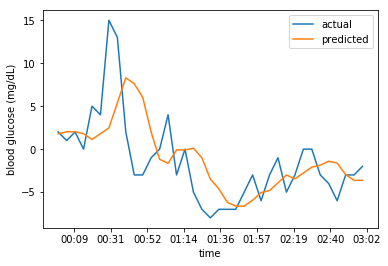In :
predicted_sgvs = testframe.iloc.sgv + np.cumsum(predicted_deltas)
plot_glucose(testframe.index, testframe.sgv, predicted_sgvs)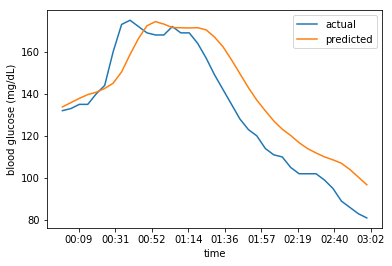We can also see how the model performs if we ask if to infer 3 hours in the future. This is not a meaningful thing to do in this context, as it does not account for insulin (or carb) deliveries during this time period, but it's nevertheless a fun visualization.

In :
def feed_dict_row_delta(row, prevdelta):
"""Construct a data dictionary for inference
from a single row and a prevdelta."""
return {
model.car: np.array([row['ca']]).reshape([-1, 1]),
model.ia: np.array([row['ia']]).reshape([-1, 1]),
model.prevdelta: np.array([prevdelta]).reshape([-1, 1]),
}

predicted_deltas = np.zeros(len(testframe))
for i in range(len(testframe)):
row = testframe.iloc[i]
if i == 0:
# Start off with the initial mean delta.
prevdelta = row['prevmeandeltasgv']
else:
prevdelta = predicted_deltas[i-1]
predicted_deltas[i] = \
sess.run(model.predicted_delta, feed_dict=feed_dict_row_delta(row, prevdelta))
predicted_sgvs = testframe.iloc.sgv + np.cumsum(predicted_deltas)
plot_glucose(testframe.index, testframe.sgv, predicted_sgvs)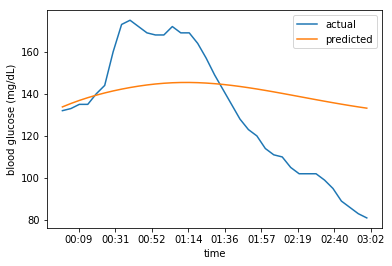## Visualizing the model by synthesizing scenarios¶

Another useful "sanity check" on our model is to see how it responds to certain common scenarios. We have certain ideas for how your body should respond to, say, eating carbohydrates without insulin, or giving a correction bolus.

The following sets up code to synthesize (future) events, and then visualize the model's predictions for how the body responds to these events.

In :
from numpy.lib.stride_tricks import as_strided

def rolldot(events, coeffs, w=W):
"""Helper function to compute a rolling dot-product,
used to compute current insulin and carbohydrate activity
according to our curves."""
events = np.append(np.zeros(w), events)
stride, = events.strides
strides = as_strided(events, shape=[len(events) - w + 1, w], strides=[stride, stride])
strides = strides[1:]
return np.dot(strides, coeffs)

def feed_dict_synthetic(carbs, boluses, prevdelta):
"""Construct a data dictionary with the
provided carb and bolus events. The returned
feed dict contains only the last entry."""
return {
model.car: rolldot(carbs, carb_coeffs['uciM'])[-1].reshape([1, 1]),
model.ia: rolldot(boluses, humalog_coeffs)[-1].reshape([-1, 1]),
model.prevdelta: np.array([prevdelta]).reshape([-1, 1]),
}

"""Infers future glucose values based on the provided
carbohydrate and bolus (insulin) events."""
prevdelta = 0
deltas = np.zeros(len(carbs))
for i in range(len(carbs)):
prevdelta = sess.run(
model.predicted_delta,
feed_dict=feed_dict_synthetic(carbs[:i+1], boluses[:i+1], prevdelta),
)
deltas[i] = prevdelta
return np.cumsum(deltas)

def plot_predict_glucose(title, events):
"""Plot the model's glucose predictions given
a set of events."""
maxtime = 0
for (time, _, _) in events:
if time > maxtime:
maxtime = time
carbs, boluses = np.zeros(maxtime+1), np.zeros(maxtime+1)
for (time, event, v) in events:
if event == 'C':
carbs[time] = v
elif event == 'B':
boluses[time] = v
else:
raise Exception("unknown event type")
glucose = predict_glucose(carbs, boluses)
n = len(glucose)
time = np.linspace(0., 5.*n, n, endpoint=False)
fig = plt.figure()
ax.set_ylabel('change in blood glucose (mg/dL)')
ax.set_xlabel('time (m)')
ax.set_title(title)
ax.plot(time, glucose)
plt.show()

plot_predict_glucose('prebolus for food', [(0, 'B', 100), (4, 'B', 2000), (6, 'C', 20)])
plot_predict_glucose('do nothing', [])
plot_predict_glucose('give big bolus', [(0, 'B', 2000)])
plot_predict_glucose('eat carbs then bolus later', [(0, 'C', 10), (4, 'B', 1000)])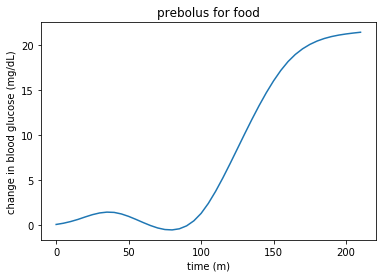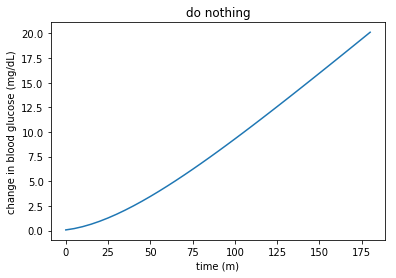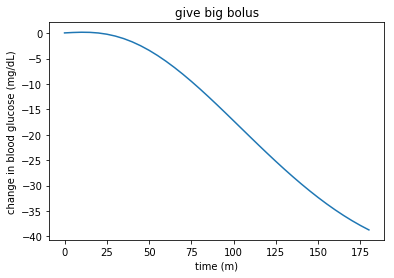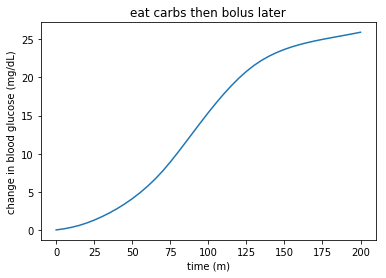As we can see, our simple model follows intuition:

• when we pre-bolus for food, glucose dips a little before rising and settling at a slightly higher level;
• if we do nothing (i.e., no insulin delivery, no carb delivery), endogenous glucose production ensures that glucose levels steadily rise;
• if we give a big bolus, glucose levels drop significantly;
• if we eat carbs before bolusing, glucose levels rise and then level off.

# Creating an insulin delivery schedule¶

We are now ready to use our blood glucose model to create an insulin delivery controller. The controller should use the predictions given by the glucose model to learn a schedule of insulin deliveries that maximizes the amount of time where glucose is in range (near the setpoint).

Here's how it's going to work: given the last 6 hours of insulin and carb deliveries, we use our model to predict the next 6 hours. While the previous 6 hours of insulin delivery are fixed immutable, we can train the schedule for the next 6 hours. We use a loss function that minimizes the (squared) distance from our desired setpoint.

In :
def roll_indices(n):
m = np.zeros([n, n], dtype=int)
for i in range(0, n):
m[i, :] = np.arange(i, i+n, dtype=int)
return m

# rolled is a W-by-W matrix of indices used to construct
# lookback windows for carb and insulin curves.
# These cannot be computed outside of Tensorflow anymore because they
# are part of the computation that is involved in optimization.
rolled = roll_indices(W)

class schedule:
# future_dpids is the next 6 hours of insulin deliveries, as learned
# by the schedule model.
future_dpids = tf.Variable(tf.zeros([W, 1]), name='future_dpids')

# These are placeholders used to feed the glucose model.
past_dpids = tf.placeholder(tf.float32, [W, 1], name='past_dpids')
past_ucis = tf.placeholder(tf.float32, [W, 1], name='past_ucis')

# Last_delta is used to initialize the pdgsv factor from the glucose model.
last_delta = tf.placeholder(tf.float32, , name='last_delta')
# Deviation is the deviation from the desired setpoint: i.e., the amount
# (up or down) glucose should move and settle to, relative to the starting
# point.
deviation = tf.placeholder(tf.float32, [], name='deviation')

dpids = tf.gather(
tf.reshape(tf.concat([past_dpids, future_dpids], 0), [-1]),
rolled,
)
ucis = tf.gather(
tf.reshape(tf.concat([past_ucis, tf.zeros([W, 1])], 0), [-1]),
rolled,
)
ia = tf.matmul(dpids, humalog_coeffs.reshape([-1, 1]))
car = tf.matmul(ucis, carb_coeffs['uciM'].reshape([-1, 1]))

egp_effect = model.egp_effect
carb_effect = car*model.carb_coeff
insulin_effect = ia*model.insulin_coeff

# Delta contains the predicted deltas, sans the prevdelta term.
# Since prevdelta uses the previous SGV delta, we must use the
# predictions yielded by the model itself to compute this term
# for future data points. We do this by scanning over the tensor
# of deltas, accumulating contributions.
delta = egp_effect + carb_effect - insulin_effect
delta = tf.scan(lambda a, x: a*model.prevdelta_coeff+x, delta, last_delta)
cumdelta = tf.scan(lambda a, x: a + x, delta)

# Define a loss function. This is the function we will optimize.
# Negative insulin deliveries are not permitted (they are not physically
# possible—it would be nice if they were!), and so we must penalize
# these heavily so that the model does not find schedules that would
# subtract insulin. (Interestingly, if it could, it could almost always
# correct positive deviations immediately, since it could give a large
# amount of insulin and then cut the tail.)
scaleneg = 100000.
loss = tf.reduce_sum(tf.pow(deviation+cumdelta, 2))/(2*W) \
- scaleneg*tf.minimum(tf.reduce_min(future_dpids), 0)

clip = future_dpids.assign(tf.maximum(future_dpids, 0.))

sess.run(future_dpids.initializer)


With a scheduling model in hand, we can go through the same exercise as above: synthesize a scenario, and see what insulin delivery schedule is learned. The big difference here is that the glucose values reflect how the model believes glucose is going to respond not just to recent history but to the insulin schedule that's been learned by the model itself.

We'll plot several scenarios: each includes both the predicted glucose schedule as well as the learned insulin delivery schedule.

In :
def schedule_insulin(ucis, dpids, deviation, niter=500, display_loss=False):
"""Create a 6-hour insulin schedule based on the past carb and insulin inputs,
correcting for the provided deviation."""
# Reset the future DPIDS back to their initial value.
sess.run(schedule.future_dpids.assign(np.zeros([W, 1])))
# Extend history to go W in the past.
feed_dict = {
schedule.past_ucis: ucis.reshape([-1, 1]),
schedule.past_dpids: dpids.reshape([-1, 1]),
schedule.last_delta: np.array([0.]).reshape(),
schedule.deviation: deviation,
}
# Learn the insulin delivery schedule.
for i in range(niter):
sess.run(schedule.learning_step, feed_dict=feed_dict)
if display_loss and i%100 == 0:
loss = sess.run(schedule.loss, feed_dict=feed_dict)
print("iter {} loss {}".format(i, loss))
dpids, cumdelta = sess.run([schedule.future_dpids, schedule.cumdelta], feed_dict=feed_dict)
return dpids, cumdelta

def plot_schedule_insulin(title, deviation, events):
"""Plot the learned insulin schedule given the provided deviation and
past events."""
ucis, dpids = np.zeros(W), np.zeros(W)
for (time, event, v) in events:
if time >= 0:
if event == 'C':
ucis[time] = v
elif event == 'B':
dpids[time] = v
else:
raise Exception("unknown event type")

dpids, cumdelta = schedule_insulin(ucis, dpids, deviation)
n = len(dpids)
time = np.linspace(0., 5.*n, n, endpoint=False)
fig, ax1 = plt.subplots()
ax2 = ax1.twinx()
ax1.plot(time, deviation+cumdelta)
ax1.set_title(title)
ax2.bar(time, dpids, width=5)
ax1.set_xlabel("minutes")
ax1.set_ylabel("deviation (mg/dL) ")
ax1.set_ylim([-40, 40])
ax2.set_ylabel("delivery (mU/5m)")
ax2.set_ylim([0, 500])
ax1.legend()
plt.show()

plot_schedule_insulin('correct deviation of 30', 30, [])
plot_schedule_insulin('correct deviation of -30', -30, [])
plot_schedule_insulin('hold steady after bolus', 0, [(-1, 'B', 400)])

/usr/local/var/pyenv/versions/3.6.1/lib/python3.6/site-packages/matplotlib/axes/_axes.py:545: UserWarning: No labelled objects found. Use label='...' kwarg on individual plots.
warnings.warn("No labelled objects found. "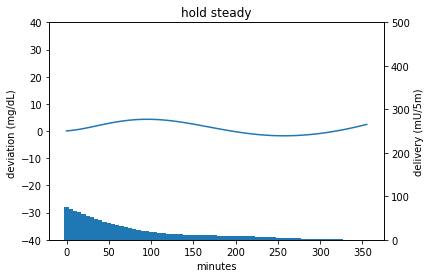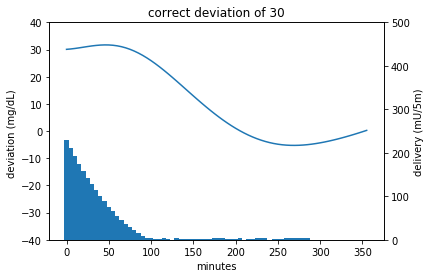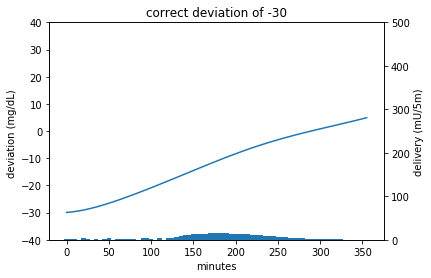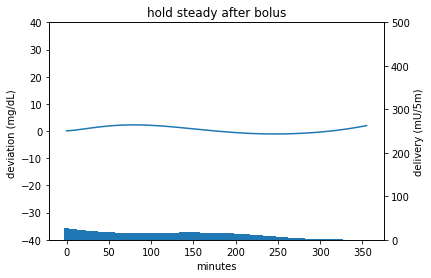We can see here that the scheduling model is able to learn a schedule that minimizes deviation from our desired setpoint (0 on the y axis). Since the model minimizes the mean-squared-error, this means that it also takes into account cumulative effects. For example, in the second scenario, the learned insulin schedule frontloads most of the insulin (and, presumably, withholds delivery later) in order to quickly bring the blood glucose down to the setpoint. Thus, the traditional distinction between "basal" and "bolus" insulin deliveries is gone: the model simply delivers insulin that maximizes the amount of time spent near the desired setpoint.

A closed-loop insulin delivery system computes a schedule at regular intervals in order to account for new inputs (insulin delivered, carbs consumed) and for unexplained deviations in the model (i.e., where the actual deviations differ from the expected ones). The system then delivers insulin according to the schedule.

# Epilogue¶

In this notebook, we have derived a (very) simple model for blood glucose, and shown how to make use of this model in an insulin controller, such as may be used by a closed-loop insulin delivery system.

At a very high level, this is the strategy that is used in tinyAP, but reality demands a much more complicated model, to account for time-related effects, glucose-related effects, and other nonlinearities.

The framework set up here is a flexible one: any modelled effect is automatically accounted for when computing insulin deliveries. For example, if we make EGP a function of time (as is done in the model used by tinyAP), time is automatically accounted for: if, in an hour, the estimated EGP increases or decreases, the learned schedule will anticipate this in good time and deliver (or withhold) insulin so that the desired setpoint is maintained. This, of course, is similar to a traditional "basal schedule", but with a key difference: Basal schedules are delivery schedules, that is, they specify the rate of insulin to be delivered at specific times, in anticipation of increased (or decreased) insulin needs in the future. With model predictive control, we instead model EGP and other effects directly; the insulin scheduler learns a delivery schedule based instead on the directly modelled effects.

Another advantage of this approach is its composability. Because we have a general framework for relating inputs (insulin, carbohydrates, time, glucose levels, etc.) to predicted blood glucose, improving the model (in the sense that it accounts for more of the observed glucose level variance) automatically improves the AP's performance. As an example, the model used by tinyAP includes parameters for both time (to account for different insulin sensitivies and endogenous glucose production rates throughout the day) and glucose levels (to account for observed effects of increased insulin resistance at higher glucose levels, presumably due to hepatic activity). In order to account for these observed effects, only the model itself was modified; the rest of the system remained unchanged.

In the next installment, we'll build on the simple model here to account for some of the aforementioned effects.